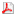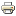Algebra seminar - October 3, 1pm, lecture room M5We will continue on Thursday, October 3, in M5 at 1pm by the talk

#### P. Arndt

Ranges of functors and geometric elementary classes

Abstract:
Given first order theories S,T and a functor F:Mod(S)-->Mod(T) between their categories of models, one can ask whether objects in the image of F satisfy first order sentences other than those of T, or whether the essential image of F can be described as Mod(T') for an extension T' of T. If Mod(S), Mod(T) are k-accessible and F is a strongly k-accessible functor for some cardinal k, we can give criteria for this in the realm of Espíndola's k-geometric first order theories.
To this end we consider k-classifying toposes associated to S and T. The hypotheses ensure that the functor F is induced by a k-geometric essential morphism between them. The criteria are then obtained by factorizing this geometric morphism appropriately. We will explain the involved notions and give examples and applications.

Last Updated on Monday, 30 September 2019 14:50